×

×

UCMERCED / Business / CHEM 002 / How can we identify dipole-dipole forces?

# How can we identify dipole-dipole forces? Description

##### Description: All Chapters Covered for the midterm #2 (5-9)
11 Pages 177 Views 1 Unlocks
Reviews

Chem 002: Study Guide #2

## How to identify dipole-dipole forces?Chapter 5:

1. VSEPR Basic Model

- The valence-shell electron-pair repulsion (VSEPR) model allows us to predict which of the possible structures is actually observed in most cases. A combination of VSEPR and a bonding model, such as Lewis electron structures, is necessary to understand the presence of multiple bonds.

1. Draw Lewis structure

2. Region of high electron density are either bonding or lone pairs which repel one another to minimize electron- electron repulsion

3. Electron pairs in multiple bonds are treated the same as single bonds

4. Molecular shape is named for where nuclei are, not electrons! The proper name of this shape is trigonal pyramidal. The central sulfur atom is above the plane of the three oxygen atoms which form a triangular base for the

## Enumerate the types of intermolecular forces.pyramid.

-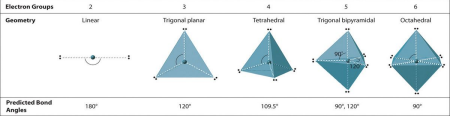2. Molecules

- a group of atoms bonded together, representing the smallest fundamental unit of a chemical compound that can take part in a chemical reaction.

- Molecules use VSEPR

- water, consisting of two hydrogen atoms and one oxygen atom (H2O), carbon dioxide, consisting of one carbon atom bonded to two oxygen atoms (CO2), and sulfuric acid, consisting of two hydrogen atoms, one sulfur atom, and four oxygen atoms (H2 SO4)

## What is reaction yield?We also discuss several other topics like How can we construct a confidence interval from a sample to estimate a population proportion?

- Valence bond theory-

- Molecular Orbital

3. Polarity

- A nonpolar molecule has no electric dipole moment. If you want to learn more check out Define enthalpy.

- Symmetry = Nonpolar

- Consequence of Molecule Shape

- Double or triple bonds fix atoms into a position.

- Bond dipole–Separation of charge based upon different

electronegativities of atoms in covalent bond

- Overall polarity of molecule–Determined by summing the

polarities of all individual bonds–Include strengths of bonds and

orientations

- Permanent dipole–Permanent separation of charge due to unequal distribution of bonding and/or lone pairs of electrons

- Dipole moment –A quantitative expression of the polarity of a

molecule –Expressed in units of debyes (D) –Where 1 D = 3.34 ×

10-30 coulomb-meter

Chapter 6:

1. Liquid structures

2. Origin of intermolecular Forces

3. London Dispersion Forces

4. Viscosity Don't forget about the age old question of What is the function of phyton?

- The viscosity of a fluid is the measure of its resistance to gradual deformation by shear stress or tensile stress. For liquids, it corresponds to the informal concept of "thickness": for example, syrup has a higher viscosity than water.

5. Interaction with Polar Molecules

- A polar molecule with two or more polar bonds must have a geometry which is asymmetric in at least one direction, so that the bond dipoles do not cancel each other. Polar molecules interact through dipole–dipole intermolecular forces and hydrogen bonds.

-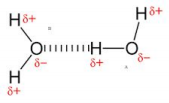If you want to learn more check out How do we have social control?

6. Dipole- Dipole Interaction

- How to Identify Dipole-Dipole Forces. Polar molecules contain polar bonds that contain form dipoles. To determine whether a bond is polar, you look at the electronegativity difference between the atoms. If the electronegativity difference is between 0.4 and 1.7, then it is considered to be a polar bond.

- Examples of Dipole-Dipole Interactions. Another example of a dipole–dipole interaction can be seen in hydrogen chloride (HCl): the relatively positive end of a polar molecule will attract the relatively negative end of another HCl molecule.

- Dipole-dipole forces are attractive forces between the positive end of one polar molecule and the negative end of another polar molecule. Dipole-dipole forces have strengths that range from 5 kJ to 20 kJ per mole. The partially positive end of a polar molecule is attracted to the partially negative end of another. 7. Ion Dipole

- An ion-dipole interaction is the result of an electrostatic interaction between a charged ion and a molecule that has a dipole. It is an attractive force that is commonly found in solutions, especially ionic compounds dissolved in polar liquids.

- Ion-dipole forces are generated between polar water molecules and a sodium ion. The oxygen atom in the water molecule has a slight negative charge and is attracted to the positive sodium ion. These intermolecular ion-dipole forces are much weaker than covalent or ionic bonds.

8. Hydrogen Bonding

- A hydrogen bond is a partially electrostatic attraction between a hydrogen (H) atom which is bound to a more electronegative atom or group, such as nitrogen (N), oxygen (O), or fluorine (F)—the hydrogen bond donor—and another adjacent atom bearing a lone pair of electrons—the hydrogen bond acceptor.If you want to learn more check out Poverty is caused by what?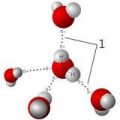9. Hydrogen bonds are particularly strong dipole−dipole interactions, so the hydrogen bonds between molecules of ethanol should be stronger than the dipole−dipole interactions between molecules of dimethyl ether. More energy is required to overcome those stronger interactions, which is why ethanol has a higher boiling point.

10. Dimethyl ether and ethanol are constitutional isomers, yet their boiling points differ by more than 100K—a difference due to the hydrogen bonding between the OH groups present in molecules of ethanol but not present in molecules of dimethyl ether. 11. Viscosity and Surface Tension Don't forget about the age old question of Who is the father of containment?

- Viscosity, on the other hand, is related to a liquid's resistance to being deformed or moved. This is caused by the friction between molecules. Compared to viscosity, surface tension is a simpler phenomenon.

12. The shape of the Meniscus

- It can be either concave or convex, depending on the liquid and the surface. ... Conversely, a convex meniscus occurs when the particles in the liquid have a stronger attraction to each other than to the material of the container. Convex

meniscus occur, for example, between mercury and glass in barometers and thermometers.

-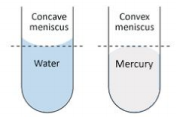13. Types of Intermolecular Forces

- London Dispersion Force.

- Dipole-Dipole Interaction.

- Ion-Dipole Interaction.

- Van der Waals Forces.

14. Phase Diagrams

- The simplest phase diagrams are pressure–temperature diagrams of a single simple substance, such as water. The axes correspond to the pressure and temperature. The phase diagram shows, in pressure–temperature space, the lines of equilibrium or phase boundaries between the three phases of solid, liquid, and gas.

15. Phase Diagram of Carbon Dioxide

- At temperature of 197.5 K (-78.5oC), the vapor pressure of solid carbon dioxide is 1 atm (760 torr). At this pressure, the liquid phase is not stable, the solid simply

sublimates. Thus solid carbon dioxide is called dry ice, because it does not go through a liquid state in its phase transition at room pressure.

-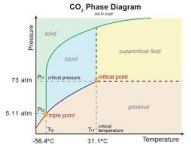16. Properties of Water

- Polarity. A water molecule is slightly charged on both ends.

- Cohesion. Hydrogen bonds hold water molecules together.

- High Specific Heat.

Chapter 7:

1. Stoichiometric Coefficients

- Stoichiometric coefficient (ν) is the number appearing before the symbol for each compound in the equation for a chemical reaction. By convention, it is negative for reactants and positive for products. Stoichiometric coefficients describe the stoichiometry of the chemical reaction.

2. Symbols in a Chemical Equation

- Aqueous, or dissolved in water – represented by lowercase aq. This is written after each reactant or product in brackets and in subscript, meaning, a bit lower than the actual text. State symbols represent the state of each reactant and each product in a reaction, and must be included in every chemical equation.

- Subscripts: Part of the chemical formulas of the reactants and products that indicate the number of atoms of the preceding element.

- Coefficient: A small whole number that appears in front of a formula in a balanced chemical equation.

3. Balancing Chemical Equations

- Write down your given equation

- Write down the number of atoms per element

- Save hydrogen and oxygen for last, as they are often on both sides - Start with single elements

- Use a coefficient to balance the single carbon atom

- Balance the hydrogen atoms next

- Balance the oxygen atoms

4. Stoichiometric Calculations

- Balance the equation.

- Convert units of a given substance to moles.

- Using the mole ratio, calculate the moles of substance yielded by the reaction. - Convert moles of wanted substance to desired units.

5. Percentage Composition

- Percent composition is the percentage by mass of each element in a compound. Examples: The percent composition of water is 20% hydrogen and 80% oxygen. - The percent composition of a compound is an expression of the elemental composition of that compound. The percent composition of each element (actually the mass percent) is equal to the mass of that element divided by the total mass present (the formula mass) multiplied by 100%.

6. Determining Molecular Formulas

- Calculate the empirical formula mass. You determine this number by finding the mass of HO (1 hydrogen atom and 1 oxygen atom).

- Divide the gram molecular mass by the empirical formula mass.

- Multiply each of the subscripts within the empirical formula by the number calculated in Step 2.

7. Combustion Analysis

- Combustion analysis is a method used in both organic chemistry and analytical chemistry to determine the elemental composition of a pure organic compound by combusting the sample under conditions where the resulting combustion products can be quantitatively analyzed.

8. Limiting Reactants and Percent Yield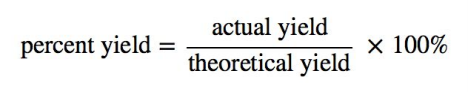- Balance the chemical equation for the chemical reaction.

- Convert the given information into moles.

- Use stoichiometry for each individual reactant to find the mass of product produced.

- The calculated or expected amount of product is called the theoretical yield. The amount of product actually produced is called the actual yield. When you divide actual yield by theoretical yield you get a decimal percentage known as the percent yield of a reaction.

9. Reaction Yield

- Yield (chemistry) The theoretical yield is the amount predicted by a stoichiometric calculation based on the number of moles of all reactants present. This calculation assumes that only one reaction occurs and that the limiting reactant reacts completely.

- Calculate the molar mass of each reactant

- Convert the amount of each reactant from grams to moles

- Find the ratio of your reactions

- Find the ideal ratio for the reaction

- Compare the ratios to find the limiting reactant.

- Actual

- Amounts of products calculated from the complete reaction of the limiting reagent is called theoretical yields, whereas the amount

actually produced of a product is the actual yield. The ratio of actual yield to theoretical yield expressed in percentage is called the percentage yield.

- Theoretical

- The theoretical yield is a term used in chemistry to describe the maximum amount of product that you expect a chemical reaction could create. You need to begin with a balanced chemical equation and define the limiting reactant.

Page ExpiredIt looks like your free minutes have expired! Lucky for you we have all the content you need, just sign up here